Assessing the Structural Complexity of Computer and Communication NetworksOnline Appendix

Andrzej Kamisiński, Piotr Chołda and Andrzej JajszczykDepartment of Telecommunications, AGH University of Science and Technology in Kraków, Poland

BibTeX EndNote

List of symbols

 $n$ Number of vertices in a graph $m$ Number of edges in a graph

Estimated structural complexity of modified variants of the Petersen graph

In the following figure, a sequence of graphs is presented which reflects some structural modifications introduced into the Petersen graph (1). Edges marked in orange are the new edges.To give an example of how different structural complexity indices react to various changes in network topology, the structural complexity of the above graphs was estimated with the aid of seventeen metrics discussed in the paper. The following table contains the results. With the exception of the Symmetry Index, the results are normalized according to the maximum value in each row. The maximum and minimum values in each row are marked in orange and blue, respectively. Please note that the Symmetry Index was included in the tables only to provide an estimation of the symmetry of a graph.

Metric123456
Complexity Index B$0.684211$$1.000000$$0.603715$$0.370279$$0.419244$$0.313317 Normalized Edge Complexity0.750000$$1.000000$$0.750000$$0.500000$$0.550000$$0.500000$
Topological Information Content$0.000000$$0.301030$$0.000000$$0.301030$$1.000000$$1.000000 Bertz Complexity Index0.500000$$0.650515$$0.500000$$0.650515$$1.000000$$1.000000$
Graph Vertex Complexity$0.546819$$0.560644$$0.779389$$0.837531$$0.828081$$1.000000 Graph Distance Complexity1.000000$$0.996486$$0.984095$$0.988215$$0.986894$$0.972804$
Medium Articulation$0.703131$$1.000000$$0.703131$$0.292547$$0.465654$$0.225772 Efficiency Complexity0.972905$$1.000000$$0.912787$$0.573986$$0.662101$$0.393782$
Graph Index Complexity$0.566838$$1.000000$$0.566838$$0.285029$$0.410915$$0.286703 Offdiagonal Complexity0.000000$$0.330830$$0.000000$$0.513459$$1.000000$$0.853384$
Spanning Tree Sensitivity (STS)$0.383707$$0.798527$$0.803221$$0.575561$$1.000000$$0.575561 Spanning Tree Sensitivity Differences (STSD)0.000000$$0.650788$$1.17 \cdot 10^{-12}$$3.13 \cdot 10^{-14}$$1.000000$$1.60 \cdot 10^{-14}$
One-Edge-Deleted Subgraph Complexity ($C_{\mathrm{1e,ST}}$)$0.142857$$1.000000$$0.857143$$0.571429$$1.000000$$0.285714 One-Edge-Deleted Subgraph Complexity (C_{\mathrm{1e,spec}})0.736842$$1.000000$$0.736842$$0.473684$$0.526316$$0.473684$
Two-Edges-Deleted Subgraph Complexity ($C_{\mathrm{2e,spec}}$)$0.550265$$1.000000$$0.550265$$0.227513$$0.285714$$0.232804 Total Walk Count (TWC)0.042041$$1.000000$$0.042041$$0.005779$$0.012526$$0.005187$
Vertex Degree Information Content$0.581121$$1.000000$$0.581121$$0.290561$$0.354664$$0.281335 Symmetry Index10.228819$$5.643856$$7.643856$$5.643856$$0.000000$$0.000000$

Structural complexity metrics — additional information

The table below presents the minimum and maximum values (or estimated lower/upper bounds, respectively) of particular metrics for simple graphs.

MetricMinimum valueMaximum value
Complexity Index B$0$$n Normalized Edge Complexity0$$0.5$
Topological Informat ion Content$0$$\log_{2}n Bertz Complexity Indexn \log_{2}n$$2n \log_{2}n$
Graph Vertex Complexity$0$$\le \log_{2}n Graph Distance Complexity0$$\log_{2} \left( n-1 \right)$
Medium Articulation$0$$1 Efficiency Complexity0$$1$
Graph Index Complexity$0$$1 Offdiagonal Complexity0$$1$
Spanning Tree Sensitivity (STS)$0$$1 Spanning Tree Sensitivity Differences (STSD)0$$1$
One-Edge-Deleted Subgraph Complexity ($C_{\mathrm{1e,ST}}$)$0$$1 One-Edge-Deleted Subgraph Complexity (C_{\mathrm{1e,spec}})0$$1$
Two-Edges-Deleted Subgraph Complexity ($C_{\mathrm{2e,spec}}$)$0$$1 Total Walk Count (TWC)0$$\infty$
Vertex Degree Information Content$0$$n \left( n-1 \right) \log_{2} \left( n-1 \right) The following table gives an intuition of the selected metrics that might be difficult to understand. Metric Formula Explanation Graph Distance Complexity R \left( \upsilon \right) = \sum_{ w = 1 }^{ e \left( \upsilon \right) } w N_{w} \left( \upsilon \right) This expression counts nodes that are at a distance w from the reference node \upsilon, multiplies these values by the corresponding distance w, and finally, provides the sum of all products. R \left( G \right) = \sum_{ i = 1 }^{ n } R \left( \upsilon_{i} \right) This expression provides the sum of R \left( \upsilon \right) values for all vertices \upsilon_{1}, \ldots, \upsilon_{n}. It will be used later as a reference in the main equation to reflect the relative "power" of particular vertices. V^{\mathrm{d}} \left( \upsilon \right) = - \sum_{ w = 1 }^{ e \left( \upsilon \right) } N_{w} \left( \upsilon \right) \frac{w}{ R \left( \upsilon \right) } \log_{2} \frac{w}{ R \left( \upsilon \right) } This function quantifies the amount of information (weighted by the N_{w} \left( \upsilon \right) values) stored in the distance-based relations for the reference vertex \upsilon. Again, weights are supposed to give special importance to terms which correspond to relatively high numbers of vertices located at certain distances w from the reference vertex. H^{\mathrm{D}} \left( G \right) = \sum_{ i = 1 }^{ n } \frac{ R \left( \upsilon_{i} \right) }{ R \left( G \right) } V^{\mathrm{d}} \left( \upsilon_{i} \right) This is the main equation in the definition of the Graph Distance Complexity metric. It provides the sum of the V^{\mathrm{d}} \left( \upsilon_{i} \right) values weighted by the related R \left( \upsilon_{i} \right) factors. Graph Vertex Complexity q_{w} \left( \upsilon \right) = \frac{ N_{w} \left( \upsilon \right) }{ n }, \quad w = 1,2,\ldots,e \left( \upsilon \right) This relation reflects the probability that an arbitrary vertex in a graph is located at a distance w from the reference vertex \upsilon. V^{\mathrm{c}} \left( \upsilon \right) = \frac{ 1 }{ n } \log_{2} n - \sum_{ w = 1 }^{ e \left( \upsilon \right) } q_{w} \left( \upsilon \right) \log_{2} q_{w} \left( \upsilon \right) Here, the amount of information stored in the probabilities q_{w} \left( \upsilon \right) is quantified (in the sum). This term has significant influence on the metric's value and is supposed to reflect the structural differences of various graphs. H^{\mathrm{V}} \left( G \right) = \frac{ 1 }{ n } \sum_{ i = 1 }^{ n } V^{\mathrm{c}} \left( \upsilon_{i} \right) This is the main equation in the definition of the Graph Vertex Complexity metric. It returns the average value of all V^{\mathrm{c}} \left( \upsilon_{i} \right) values. Offdiagonal Complexity c_{xy} = \sum_{ i = 1 }^{ n } \sum_{ j = 1 }^{ n } a_{ij} \delta_{x,a_{i}} \delta_{y,a_{j}} H \left( a_{i} - a_{j} \right) In this relation, the selected entries a_{ij} of the adjacency matrix of a given graph are added to each other, provided that the degrees of the corresponding vertices (a_{i} and a_{j}) are such that a_{i} = x \le y = a_{j}. b_{u} = \sum_{ t = 1 }^{ a_{\mathrm{max}} - u } c_{t,t+u} This expression returns the sum of the selected offdiagonal elements of the \left[ c_{xy} \right] matrix, depending on the parameter u. \tilde{b}_{u} = \frac{ b_{u} }{ \sum_{ t = 0 }^{ a_{\mathrm{max}} - 1 } b_{t} } Here, the value of b_{u} is expressed as a fraction of all b_{t} values. The greater the value, the more important the term becomes in the main equation. \mathit{OdC} \left( G \right) = - \frac{ \sum_{ u = 0 }^{ a_{\mathrm{max}} - 1 } \tilde{b}_{u} \log \tilde{b}_{u} }{ \log \left( n - 1 \right) } This is the main equation in the definition of the Offdiagonal Complexity metric. It quantifies the normalized information stored in the \tilde{b}_{u} values, which is supposed to reflect the structural differences of various graphs. Spanning Tree Sensitivity s_{ij} = N_{\mathrm{ST}} - N_{\mathrm{1e,ST}} This formula provides the difference between the number of various spanning trees in the original graph G and the corresponding number of spanning trees after the edge \upsilon_{i} \rightarrow \upsilon_{j} is removed from G. S_{ij} = s_{ij} - \left( \min \left\{ s_{ij} \right\} - 1 \right) This relation compares the s_{ij} value corresponding to the edge \upsilon_{i} \rightarrow \upsilon_{j} with the minimum value among all the s_{ij} entries determined for all edges in a given graph. w_{l} = \frac{ S_{ij}^{l} }{ \sum_{ t=1 }^{ k } S_{ij}^{t} } Here, the value of S_{ij}^{l} is expressed as a fraction of all S_{ij}^{t} values where t = 1, \ldots, k. H \left( \left\{ S_{ij} \right\} \right) = - \sum_{l=1}^{k} w_{l} \log_{2} w_{l} This expression quantifies the amount of information stored in the list of k different w_{l} entries. \mathit{STS} \left( G \right) = \frac{ H \left( \left\{ S_{ij} \right\} \right) }{ m_{\mathrm{cu}} } = \frac{ H \left( \left\{ S_{ij} \right\} \right) }{ \log \left( n^{1.68} - 10 \right) } This is the main equation in the definition of the Spanning Tree Sensitivity metric. It provides a normalized value of H \left( \left\{ S_{ij} \right\} \right). Evaluation of the selected metrics on different elementary network topologies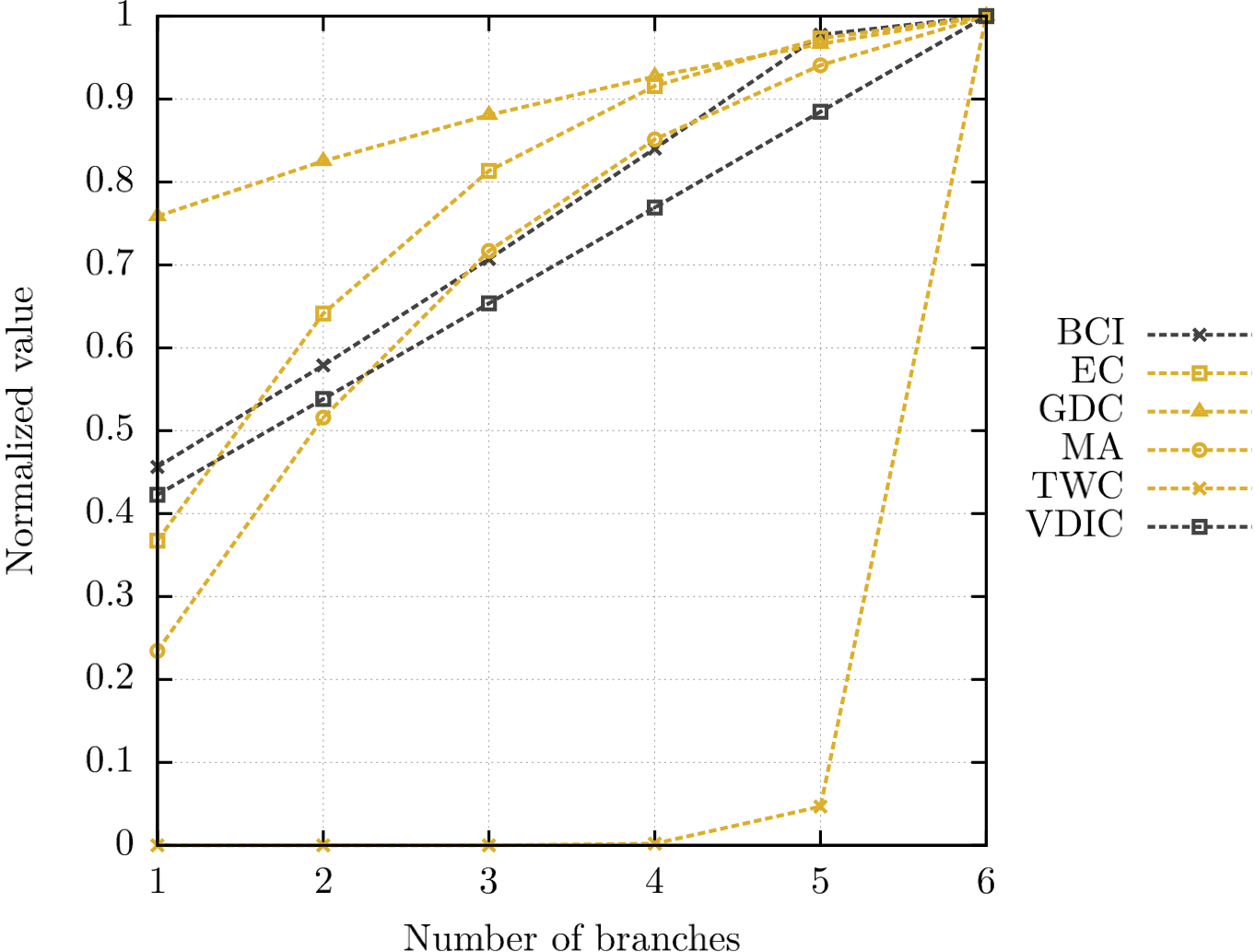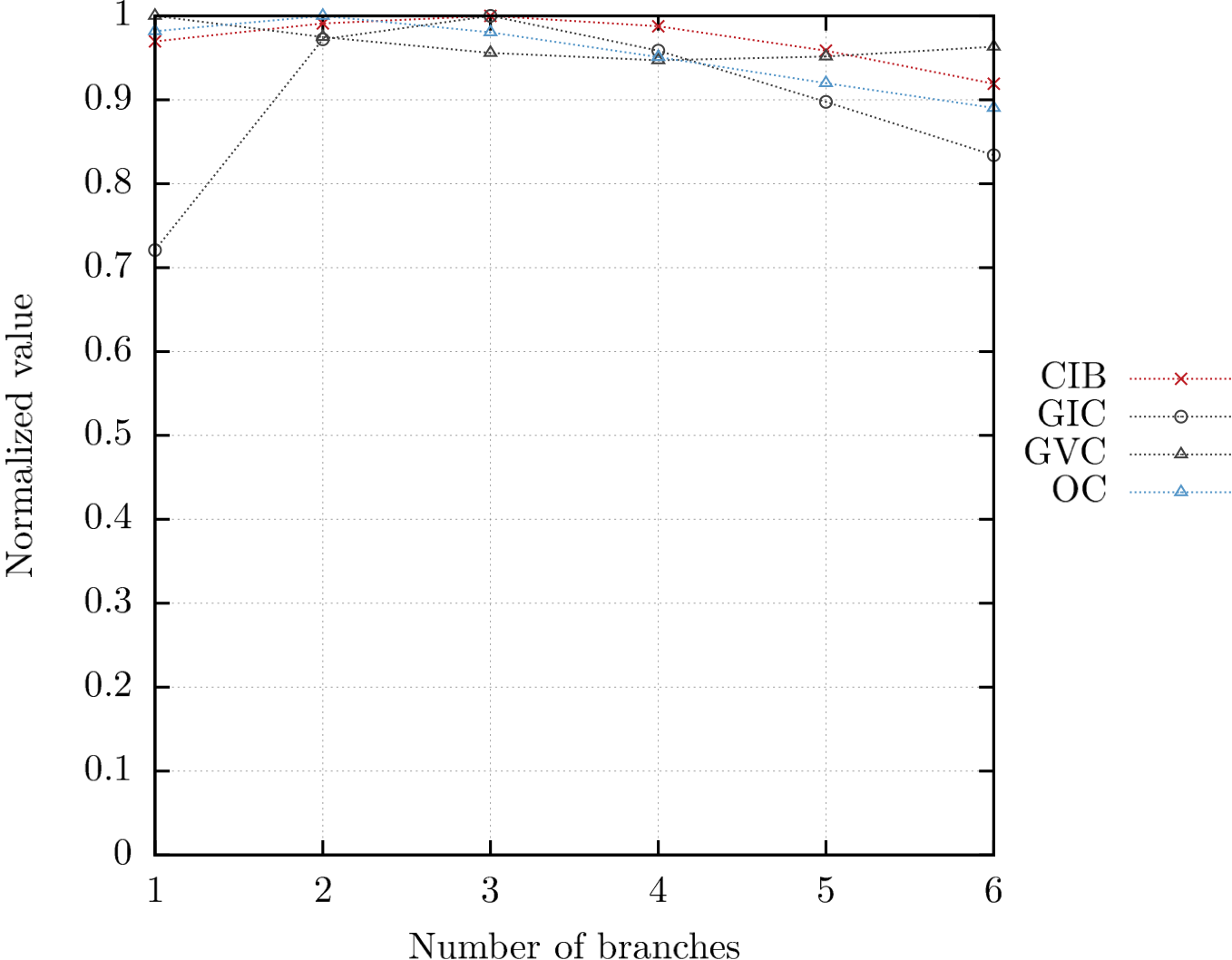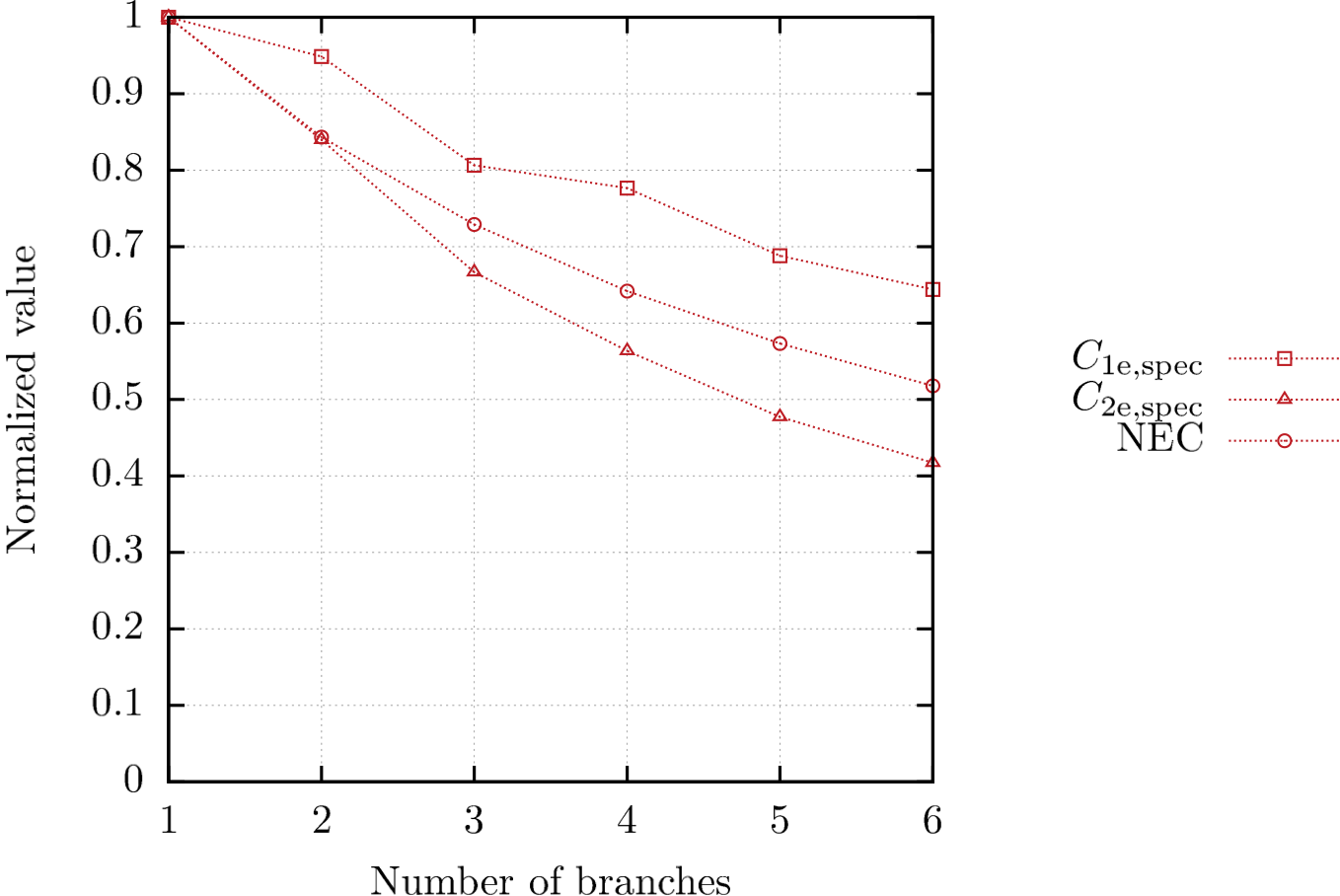The structural complexity of a tree containing variable number of identical branches, estimated with the aid of the selected metrics (BCI: Bertz Complexity Index, CIB: Complexity Index B, EC: Efficiency Complexity, GDC: Graph Distance Complexity, GIC: Graph Index Complexity, GVC: Graph Vertex Complexity, MA: Medium Articulation, NEC: Normalized Edge Complexity, OC: Offdiagonal Complexity, TIC: Topological Information Content, VDIC: Vertex Degree Information Content).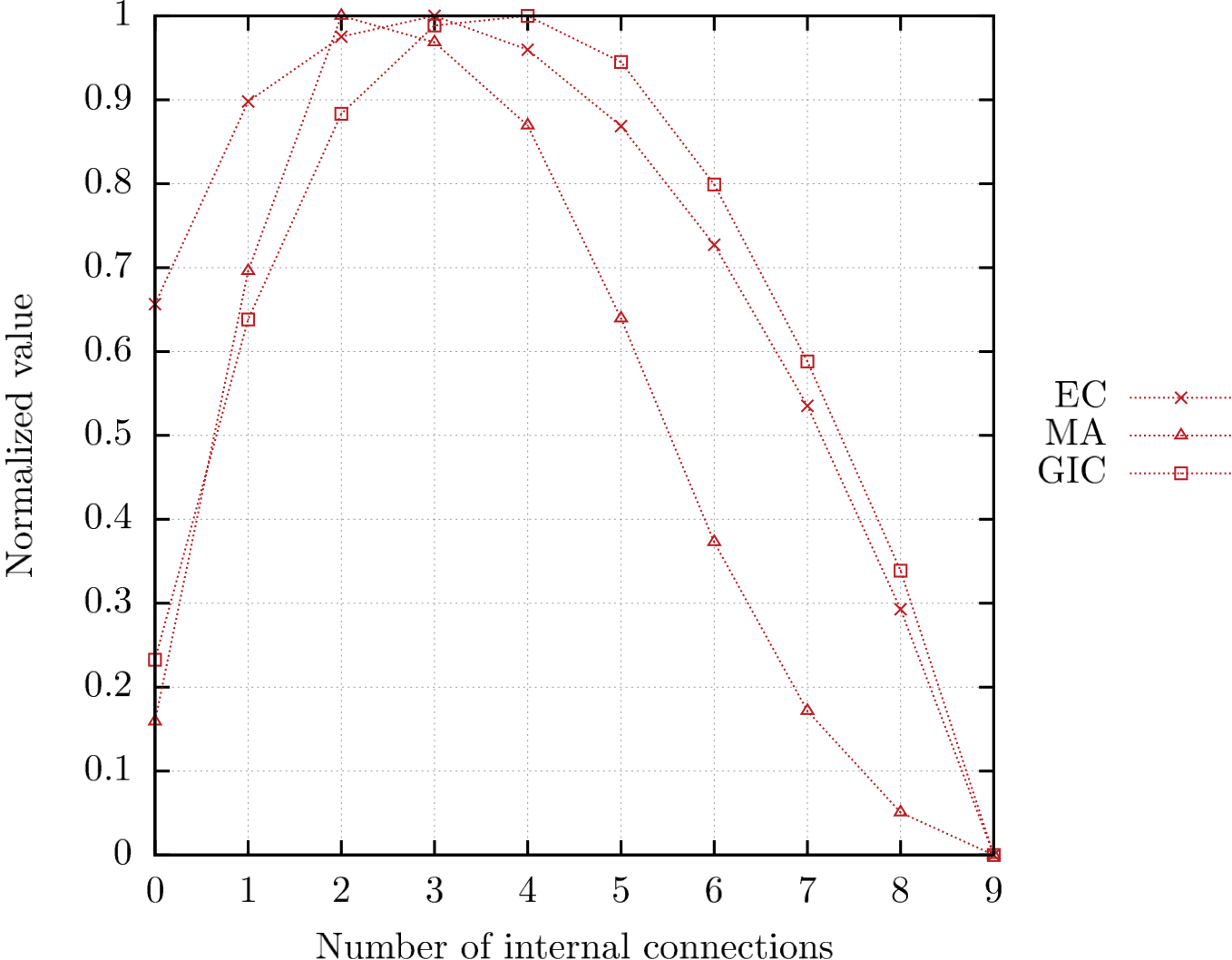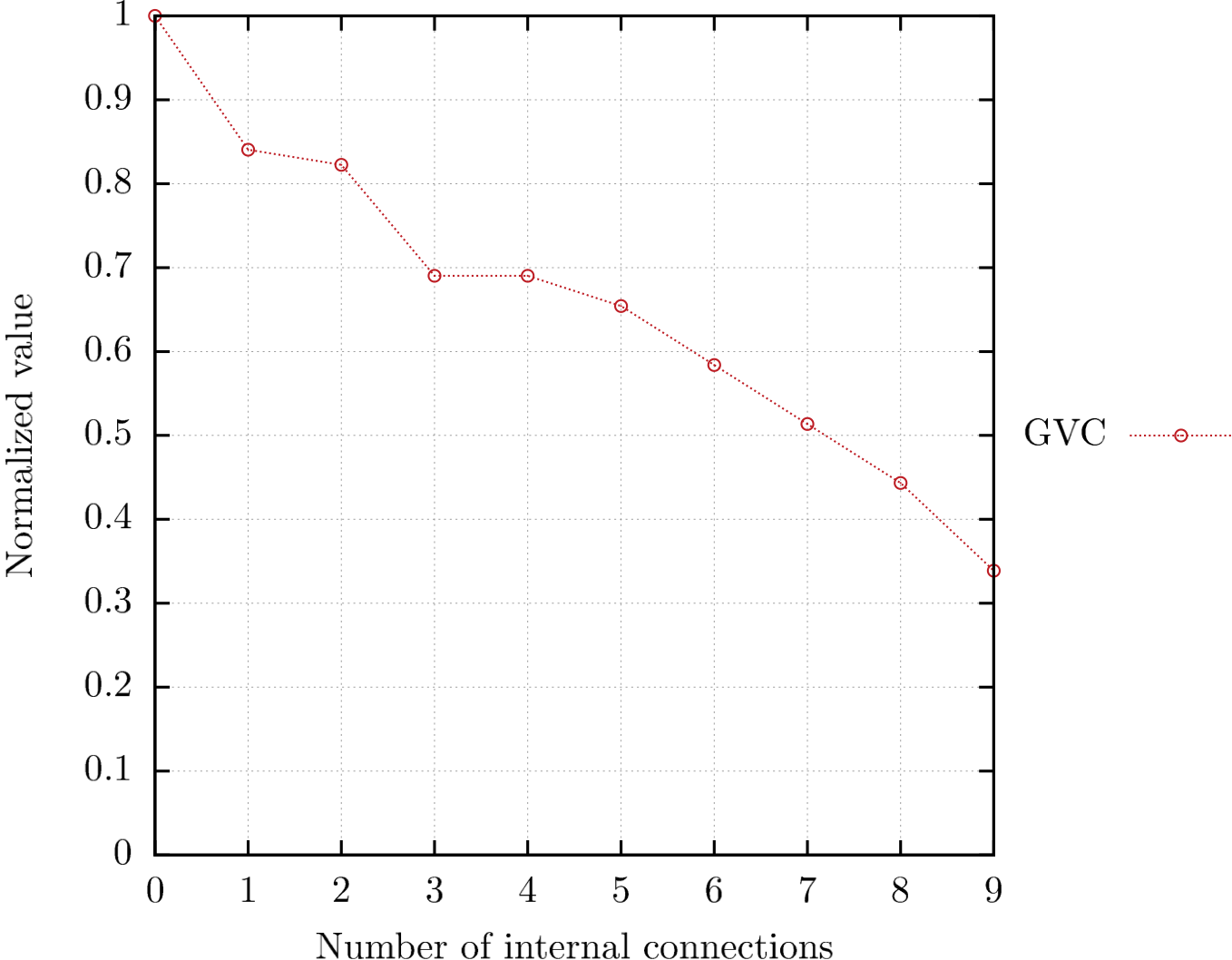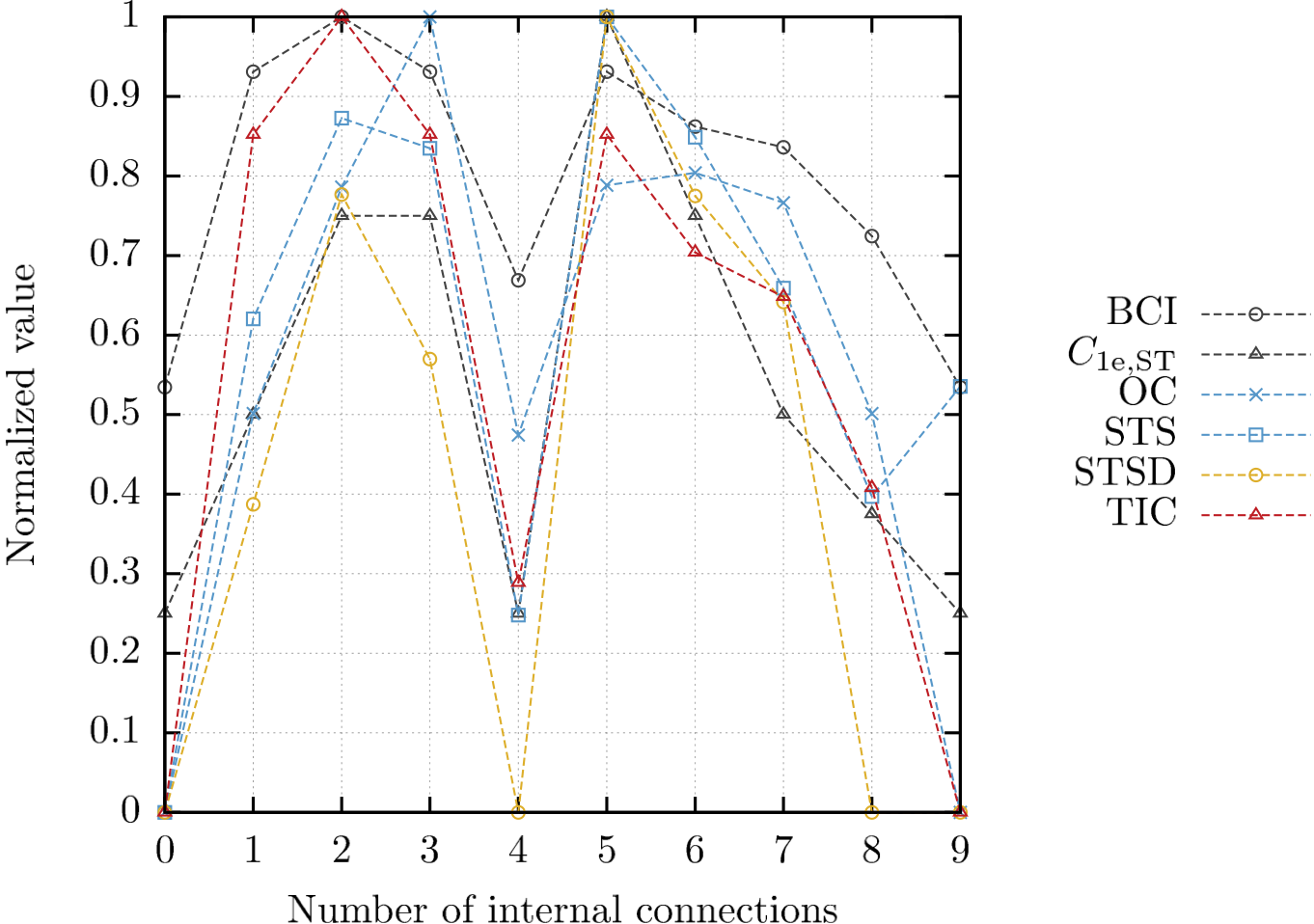The structural complexity of a ring topology with variable number of internal connections, estimated with the aid of the selected metrics (BCI: Bertz Complexity Index, CIB: Complexity Index B, EC: Efficiency Complexity, GDC: Graph Distance Complexity, GIC: Graph Index Complexity, GVC: Graph Vertex Complexity, MA: Medium Articulation, NEC: Normalized Edge Complexity, OC: Offdiagonal Complexity, TIC: Topological Information Content, VDIC: Vertex Degree Information Content). Sensitivity of the selected metrics to symmetry of network topologies with equal n, m parameters The following figure presents a sequence of graphs (all containing equal number of vertices and edges) that differ from each other with regard to their structure. The first graph in the sequence was designed to have relatively low symmetry. Then, in each of the following graphs, an existing subgraph is replaced with a fixed pattern (marked in orange) to gradually increase the symmetry. Finally, the structural complexity of all graphs in the sequence is assessed using the indices described in the paper. The results are shown in the table below. The maximum and minimum values in each row are marked in orange and blue, respectively. Please note that the ordering of graphs determined by the Symmetry Index corresponds to the figure.Metric123456 Complexity Index B0.424486$$0.470968$$0.597755$$0.633315$$0.961633$$1.000000$
Normalized Edge Complexity$1.000000$$1.000000$$1.000000$$1.000000$$1.000000$$1.000000 Topological Information Content1.000000$$0.958779$$0.900321$$0.841863$$0.517982$$0.476761$
Bertz Complexity Index$1.000000$$0.980403$$0.952610$$0.924818$$0.770838$$0.751240 Graph Vertex Complexity1.000000$$0.950518$$0.856141$$0.823494$$0.626327$$0.611009$
Graph Distance Complexity$0.978780$$0.980999$$0.985738$$0.986213$$0.999448$$1.000000 Medium Articulation0.217176$$0.291229$$0.424871$$0.553027$$0.908466$$1.000000$
Efficiency Complexity$0.432879$$0.497984$$0.652624$$0.707039$$0.968446$$1.000000 Graph Index Complexity0.655943$$0.655944$$0.681614$$0.735024$$0.965664$$1.000000$
Offdiagonal Complexity$0.955559$$1.000000$$0.928890$$0.838328$$0.818293$$0.641265 Spanning Tree Sensitivity (STS)1.000000$$0.819634$$0.912723$$0.881687$$0.845107$$0.786225$
Spanning Tree Sensitivity Differences (STSD)$1.000000$$1.000000$$0.970951$$0.970951$$0.811278$$0.811278 One-Edge-Deleted Subgraph Complexity (C_{\mathrm{1e,ST}})1.000000$$0.700000$$0.850000$$0.650000$$0.550000$$0.450000$
One-Edge-Deleted Subgraph Complexity ($C_{\mathrm{1e,spec}}$)$0.981481$$1.000000$$1.000000$$1.000000$$0.981481$$0.981481 Two-Edges-Deleted Subgraph Complexity (C_{\mathrm{2e,spec}})1.000000$$0.998617$$0.997234$$0.995159$$0.995159$$0.993776$
Total Walk Count (TWC)$0.000040$$0.000041$$0.000145$$0.000694$$0.419902$$1.000000 Vertex Degree Information Content0.752804$$0.781834$$0.828438$$0.869135$$0.973612$$1.000000$
Symmetry Index$15.616064$$22.737948$$33.271663$$43.805378$$60.308405$$67.430289 Sensitivity of the selected metrics to the number of occurrences of a fixed pattern in network topologies with equal n, m parameters The following figure presents a similar sequence of graphs (all containing equal number of vertices and edges) that differ from each other with regard to their structure. The first graph in the sequence was designed to have relatively low symmetry and no significant (large) reoccurring patterns. In each of the following graphs, the number of occurrences of a fixed pattern (marked in orange) is increased by one, while preserving the initial number of vertices and edges. Unlike the case described in the previous section, the subgraphs are replaced in a way that does not lead to a related significant increase of symmetry. The results are compared in the table below. The maximum and minimum values in each row are marked in orange and blue, respectively.Metric12345678910 Complexity Index B0.800034$$0.731279$$0.875293$$0.947298$$0.933175$$0.923125$$0.966467$$0.955565$$0.995071$$1.000000$
Normalized Edge Complexity$1.000000$$1.000000$$1.000000$$1.000000$$1.000000$$1.000000$$1.000000$$1.000000$$1.000000$$1.000000 Topological Information Content0.949052$$0.981434$$0.974694$$0.967955$$0.977238$$0.970499$$0.977238$$0.977238$$0.990717$$1.000000$
Bertz Complexity Index$0.975113$$0.990931$$0.987639$$0.984347$$0.988881$$0.985589$$0.988881$$0.988881$$0.995465$$1.000000 Graph Vertex Complexity0.956427$$1.000000$$0.939291$$0.888024$$0.897941$$0.898694$$0.893133$$0.892117$$0.874145$$0.874278$
Graph Distance Complexity$0.990185$$0.988264$$0.994023$$0.998263$$0.998381$$0.998578$$0.998580$$0.999270$$0.999935$$1.000000 Medium Articulation1.000000$$0.724168$$0.875074$$0.875074$$0.778689$$0.752275$$0.648374$$0.623876$$0.675260$$0.650345$
Efficiency Complexity$0.829679$$0.716305$$0.884078$$0.948408$$0.921345$$0.902680$$0.959412$$0.939675$$0.993661$$1.000000 Graph Index Complexity1.000000$$0.791925$$0.793251$$0.793280$$0.793264$$0.792947$$0.573633$$0.553048$$0.546155$$0.548464$
Offdiagonal Complexity$1.000000$$0.914305$$0.934438$$0.934438$$0.914305$$0.859540$$0.740034$$0.737216$$0.753757$$0.700602 Spanning Tree Sensitivity (STS)0.937577$$0.889817$$0.889817$$0.863419$$0.834996$$0.889817$$0.878677$$0.929525$$0.952950$$1.000000$
Spanning Tree Sensitivity Differences (STSD)$0.808809$$0.808809$$0.808809$$0.808809$$0.808809$$0.808809$$0.742726$$0.803980$$1.000000$$1.000000 One-Edge-Deleted Subgraph Complexity (C_{\mathrm{1e,ST}})0.909091$$0.818182$$0.909091$$0.818182$$0.727273$$0.727273$$0.772727$$1.000000$$0.863636$$0.818182$
One-Edge-Deleted Subgraph Complexity ($C_{\mathrm{1e,spec}}$)$0.981481$$0.981481$$0.981481$$0.981481$$0.981481$$0.981481$$1.000000$$0.981481$$1.000000$$1.000000 Two-Edges-Deleted Subgraph Complexity (C_{\mathrm{2e,spec}})1.000000$$0.997925$$0.999308$$0.998617$$0.996542$$0.996542$$0.997925$$0.996542$$0.995851$$0.997925$
Total Walk Count (TWC)$1.000000$$0.014414$$0.017107$$0.017517$$0.017230$$0.016496$$0.000433$$0.000269$$0.000246$$0.000260 Vertex Degree Information Content1.000000$$0.964947$$0.984668$$0.984668$$0.972245$$0.968736$$0.954419$$0.950909$$0.958208$$0.954699$
Symmetry Index$15.616064$$9.531217$$10.568254$$11.605291$$9.969312$$11.006349$$9.969312$$9.969312$$8.895238$$7.259259 Evaluation of the selected metrics on different real network topologies All six network topologies presented below have been prepared based on the data kindly shared by the Internet Topology Zoo project (http://topology-zoo.org). The selected graphs are diverse, ranging from a tree to a graph in which all vertices have degrees equal or greater than 2. They also differ in the number of elements — the largest one contains 158 vertices and 189 edges. To give more examples of how the selected structural complexity indices differentiate real network topologies modeled as simple graphs, the structural complexity of the graphs was assessed with the aid of seventeen metrics discussed in the paper. The following table contains the results. The maximum and minimum values in each row are marked in orange and blue, respectively.ARN(n=30, m=29) Tinet(n=53, m=89) TW Telecom(n=71, m=115)ULAKNET(n=82, m=82) US Carrier(n=158, m=189) Viatel(n=88, m=92) MetricARNTinetTW TelecomULAKNETUS CarrierViatel Complexity Index B0.806443$$0.774287$$0.871486$$1.000000$$0.188432$$0.149635$
Normalized Edge Complexity$1.000000$$0.983293$$0.707988$$0.378469$$0.234959$$0.368695 Topological Information Content0.335581$$0.788641$$0.836719$$0.248194$$1.000000$$0.882657$
Bertz Complexity Index$0.095835$$0.263802$$0.377187$$0.291266$$1.000000$$0.492094 Graph Vertex Complexity0.363494$$0.611230$$0.506032$$0.227848$$0.974823$$1.000000$
Graph Distance Complexity$0.674700$$0.781742$$0.850441$$0.887010$$1.000000$$0.879911 Medium Articulation0.751272$$0.579336$$0.562899$$1.000000$$0.062713$$0.005829$
Efficiency Complexity$0.822024$$0.696258$$0.764118$$1.000000$$0.294955$$0.168703 Graph Index Complexity0.941617$$0.810029$$0.671044$$1.000000$$0.094653$$0.057115$
Offdiagonal Complexity$0.817930$$0.927331$$1.000000$$0.552903$$0.446141$$0.202349 Spanning Tree Sensitivity (STS)0.197502$$1.000000$$0.978500$$0.339115$$0.859273$$0.713395$
Spanning Tree Sensitivity Differences (STSD)$0.000000$$1.000000$$0.939432$$4.86 \cdot 10^{-14}$$0.861059$$0.266706 One-Edge-Deleted Subgraph Complexity (C_{\mathrm{1e,ST}})0.073939$$1.000000$$0.726871$$0.026492$$0.216875$$0.182210$
One-Edge-Deleted Subgraph Complexity ($C_{\mathrm{1e,spec}}$)$0.816685$$1.000000$$0.788340$$0.438932$$0.336962$$0.437625 Two-Edges-Deleted Subgraph Complexity (C_{\mathrm{2e,spec}})0.684442$$1.000000$$0.620261$$0.192911$$0.113009$$0.191590$
Total Walk Count (TWC)$8.54 \cdot 10^{-59}$$7.18 \cdot 10^{-40}$$2.34 \cdot 10^{-25}$$8.76 \cdot 10^{-4}$$1.00 \cdot 10^{0}$$1.52 \cdot 10^{-43} Vertex Degree Information Content0.199343$$0.681118$$0.896818$$0.849872$$1.000000$$0.393217$
Symmetry Index$49.915733$$1.037736$$3.112676$$275.282454$$5.088608$$0.090909$

Based on the results, the following observations were made:

• The Tinet backbone network was recognized by five metrics (STS, STSD, $C_{\mathrm{1e,ST}}$, $C_{\mathrm{1e,spec}}$ and $C_{\mathrm{2e,spec}}$) to have the largest structural complexity among all the six considered network topologies. The estimated symmetry of the graph is low, while the number of elements falls within the medium range. It should be noted that the network contains numerous cycles, hence the relatively high numbers of different subgraphs or spanning trees counted by the indices. Further, the TW Telecom network has a similar structure and its estimated structural complexity was also relatively high for the same set of metrics. At the same time, the ARN network was assigned the minimum values by the STS and STSD indices, because the corresponding graph is a tree. This example shows that some of the metrics presented in the paper might be used to estimate the improvement of network reliability related to the increased redundancy in the physical network topology.
• The US Carrier network was also classified by five metrics to have the most complex structure. However, this may result mainly from the significantly higher number of elements and relatively low symmetry (compared to the other considered networks).
• The structures of the ARN and Viatel networks were classified mostly as simple. In this context, the low number of elements, high symmetry and multiple occurrences of a single pattern (star) in the ARN network seem to agree with the relation reflected in the general model that was proposed in the paper. On the other hand, The Viatel network contains considerably more elements and its estimated symmetry is lower than for the ARN network. Thus, according to the model, the number of occurrences of a fixed pattern should be the dominant factor that simplifies the description of the graph. In fact, this may be a reasonable explanation if we consider a linear topology as the fixed pattern.
• The highest and lowest estimated symmetry corresponds to the ULAKNET and Viatel networks, respectively.

Tools

To make the computation of the structural complexity easier, we decided to make our tools available to the entire research community. The tools are combined with an example ready-to-use data set which may be quickly adapted to the needs of different users. Download link: structural_complexity_tools.zip.

Once you have downloaded and unpacked the file, you will see two directories:

• data_set/ (the top-level directory containing different user-defined groups of network topologies, e.g., example_topologies),
• tools/ (containing the scripts).
The example subset (data_set/example_topologies/) includes two different network topologies (1/ and 2/). To analyze any of them with the aid of the provided tools, simply set the corresponding path as the value of constant DATA_SET_PATH in the tools/run.sh script, which is the main file used for computation and data collection. You may have to modify the other constants as well, depending on your local configuration.

Please note that the R script compute_complexity.r depends on the following packages:

• igraph,
• QuACN,
• graph,
• RBGL,
• combinat,
• Rmpfr,
• gmp,
• grid.
You will have to install the missing packages manually before using the provided R script.

The other two files in the tools/ directory (additional_metrics.py and compute_additional_metrics.py) contain our implementation of different structural complexity metrics and the code which loads network data and performs the computation. The second script is started by the tools/run.sh file. However, it may be also used directly by the user (command: python compute_additional_metrics.py).

To start the main script on Linux/Unix operating systems, please enter the following commands in the terminal (we assume you are the owner of the file):
cd tools/
chmod u+x run.sh
./run.sh
The output of the script (including all error messages) is stored in the output.log file.